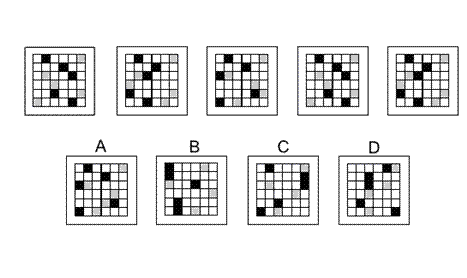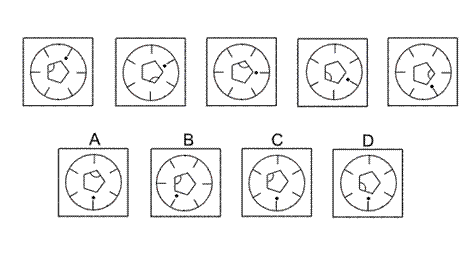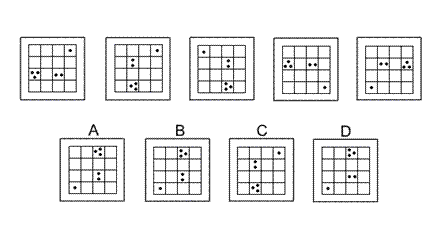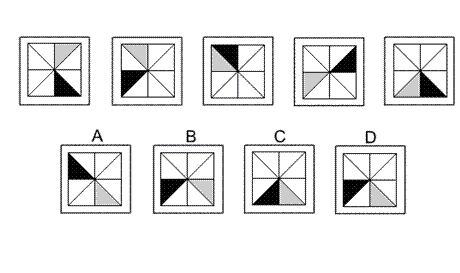Aptitude Tests 4 Me

Non Verbal Logic

Detailed solution

12.Solution: A Explanation: In this question some of the shaded squares move along the rows of the grid. The shaded squares in the first and second rows stay the same throughout. Most of the shaded squares in the third, fourth, fifth and sixth rows move two places to the left each time, until they get to the far left end when they re-emerge on the right side. This is true for all the shaded squares in rows 3, 4, 5 and 6 except for a rogue light grey square on the third row. Following these rules, the correct answer is A.

13.Solution: C Explanation: In this question there is a circular dial with a pentagon at its centre. Each rotates about the centre according to its own rule. The dial rotates clockwise by 30° each time. Following this rule, the correct answer could be A, C or D. The pentagon rotates by 144° anticlockwise each time. Following this rule, the correct answer must be C.

14.Solution: B Explanation: In this question, the grid is reflected alternately in two different axes. The first axis is along the diagonal from the top right corner of the grid to the bottom left corner of the grid. The second axis is the vertical line through the centre of the grid. Notice how the orientation of the dots changes when they are reflected. To obtain the next diagram of the sequence, the first reflection must be applied. The correct answer, therefore, is B.

15.Solution: D Explanation: In this question there is a black triangle and a grey triangle that can occupy one of each of eight triangles in the grid. The black triangle advances by two places clockwise each time - which is equivalent to a 90° clockwise turn. The grey triangle advances by one place anticlockwise each time. Following these rules simultaneously, the correct answer is D.

 1 2 3 4 5 6 7 8 9 10 11 12 13 14 15 16 17 18 19 20 21 22 23 24 25 26 27 28 29 30 31 32 33 34 35 36 37 38 39 40 41 42 43 44 45 46 47 48 49 50 51 52 53 54 55 56 57 58 59 60 61 62 63 64 65 66 67 68 69 70 71 72 73 74 75 76 77 78 79 80 81 82 83 84 85 86 87 88Passage ReadingVerbal LogicNon Verbal LogicNumerical LogicData InterpretationReasoningAnalytical AbilityBasic NumeracyAbout UsContactPrivacy PolicyMajor TestsFAQ